# Competition Level Test: Relations And Functions- 1

## 30 Questions MCQ Test Mathematics For JEE | Competition Level Test: Relations And Functions- 1

Description
This mock test of Competition Level Test: Relations And Functions- 1 for JEE helps you for every JEE entrance exam. This contains 30 Multiple Choice Questions for JEE Competition Level Test: Relations And Functions- 1 (mcq) to study with solutions a complete question bank. The solved questions answers in this Competition Level Test: Relations And Functions- 1 quiz give you a good mix of easy questions and tough questions. JEE students definitely take this Competition Level Test: Relations And Functions- 1 exercise for a better result in the exam. You can find other Competition Level Test: Relations And Functions- 1 extra questions, long questions & short questions for JEE on EduRev as well by searching above.
QUESTION: 1

### The domain of the function f(x)=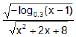is

Solution: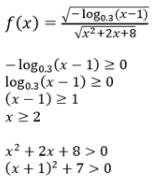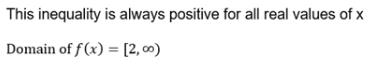QUESTION: 2

Solution:
QUESTION: 3

### If q2 – 4pr = 0, p > 0, then the domain of the function, f(x) = log (px3 + (p + q) x2 + (q + r) x + r) is

Solution: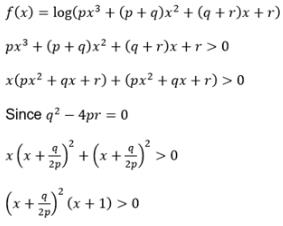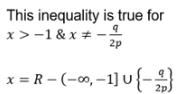QUESTION: 4

Find domain of the function f(x) =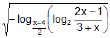Solution: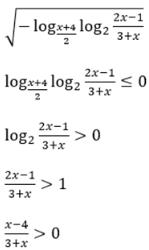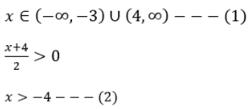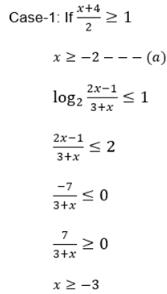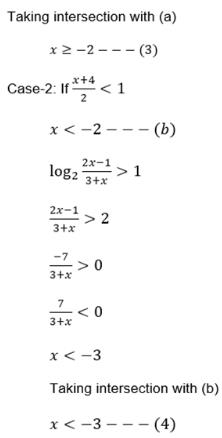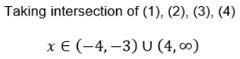QUESTION: 5

The domain of the function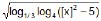is (where [x] denotes greatest integer function)

Solution: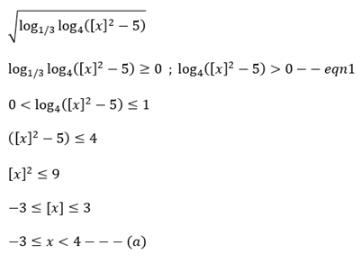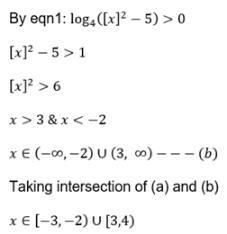QUESTION: 6

Range of f(x) = 4x + 2x + 1 is

Solution:
QUESTION: 7

Range of f(x) = log√5 {√2 (sin x –cos x) + 3} is

Solution:
QUESTION: 8

The range of the functin f(x) = log√2(2– log2 (16 sin2 x + 1)) is

Solution:
QUESTION: 9

Range of the function f(x)=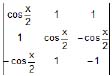is

Solution: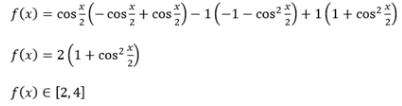QUESTION: 10

If f(x) =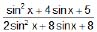, then range of f(x) is

Solution:
QUESTION: 11

The sum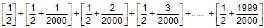is equal to (where [*] denotes the greatest integer function)

Solution: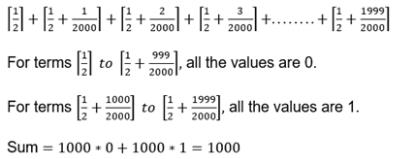QUESTION: 12

Which of the following represents the graph of f(x) = sgn ([x + 1])

Solution:
QUESTION: 13

If f(x) = 2 sin2q+4 cos (x+q) sin x. sin q+cos (2x+2q) then value of f2(x) + f2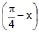is

Solution:
QUESTION: 14

Let f(x) = ax2 + bx + c, where a, b, c are rational and f : Z → Z, where Z is the set of integers. Then a+ b is

Solution:

f : Z → Z
f(x) = ax2 + bx + c
x=0, f(0) = a(0)2 + b(0) + c
= c [it is an integer]
x=1, f(1) = a + b+ c should be an integer
a + b+ c = 1
a + b = 1-c
a+b should be an integer.

QUESTION: 15

Which one of the following pair of functions are identical ?

Solution:
QUESTION: 16

The function f : [2, ∞) → Y defined by f(x) = x2 – 4x + 5 is both one–one & onto if

Solution:
QUESTION: 17

Let f : R → R be a function defined by f(x) =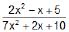then f is

Solution:
QUESTION: 18

Let f : R → R be a function defined by f(x) = x3 + x2 + 3x + sin x. Then f is

Solution:
QUESTION: 19

If f(x) =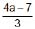x3 + (a – 3) x2 + x + 5 is a one–one function, then

Solution:
QUESTION: 20

The graph of the function y = f(x) is symmetrical about the line x = 2, then-

Solution:
QUESTION: 21

The function f : R → R defined by f(x) = 6x + 6|x| is

Solution:
QUESTION: 22

Let `f' be a function from R to R given by f(x) =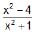. Then f(x) is

Solution:
QUESTION: 23

If f(x) = cot-1 x : R+ →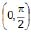and g(x) = 2x – x2 : R → R. Then the range of the function f(g(x)) wherever define is

Solution:

g(x) = 2x-x2
x(2-x)
-b/2a =. -2/(-2)
=> 1
x implies (0,2)
g(x) implies (0,1]
f(g(x)) = [π/4, π/2)

QUESTION: 24

Let g(x) = 1 + x – [x] and f(x) =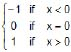, then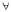x, fog(x) equals (where [*] represents greatest integer function).

Solution:
QUESTION: 25

Let f: [0, 1] → [1, 2] defined as f(x) = 1 + x and g : [1, 2] → [0, 1] defined as g(x) = 2 – x then the composite function gof is

Solution:
QUESTION: 26

Let f & g be two functions both being defined from R → R as follows f(x) =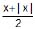and g(x) =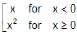. Then

Solution: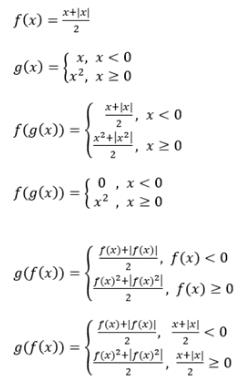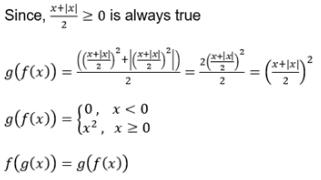QUESTION: 27

If y = f (x) satisfies the condition f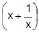= x2 +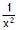(x > 0) then f(x) equals

Solution:
QUESTION: 28

The function f(x) = log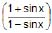is

Solution:
QUESTION: 29

It is given that f(x) is an even function and satisfy the relation f(x) =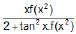then the value of f(10) is

Solution: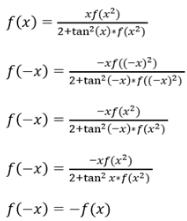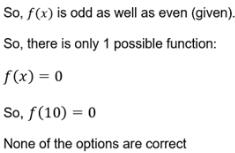QUESTION: 30

Fundamental period of f(x) = sec (sin x) is

Solution: